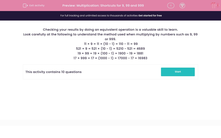# Multiplication: Shortcuts for 9, 99 and 999

In this worksheet, students develop their mental arithmetic skills by using shortcuts to help them multiply quickly and accurately.This content is premium and exclusive to EdPlace subscribers.Key stage:  KS 2

Curriculum topic:   Maths and Numerical Reasoning

Curriculum subtopic:   Mixed Problems

Difficulty level:#### Worksheet Overview

Checking your results by doing an equivalent operation is a valuable skill to learn.

Look carefully at the following to understand the method used when multiplying by numbers such as 9, 99 or 999.

11 × 9 = 11 × (10 - 1) = 110 - 11 = 99

521 × 9 = 521 × (10 - 1) = 5210 - 521 = 4689

19 × 99 = 19 × (100 - 1) = 1900 - 19 = 1881

17 × 999 = 17 × (1000 - 1) = 17000 - 17 = 16983

### What is EdPlace?

We're your National Curriculum aligned online education content provider helping each child succeed in English, maths and science from year 1 to GCSE. With an EdPlace account you’ll be able to track and measure progress, helping each child achieve their best. We build confidence and attainment by personalising each child’s learning at a level that suits them.

Get started••••